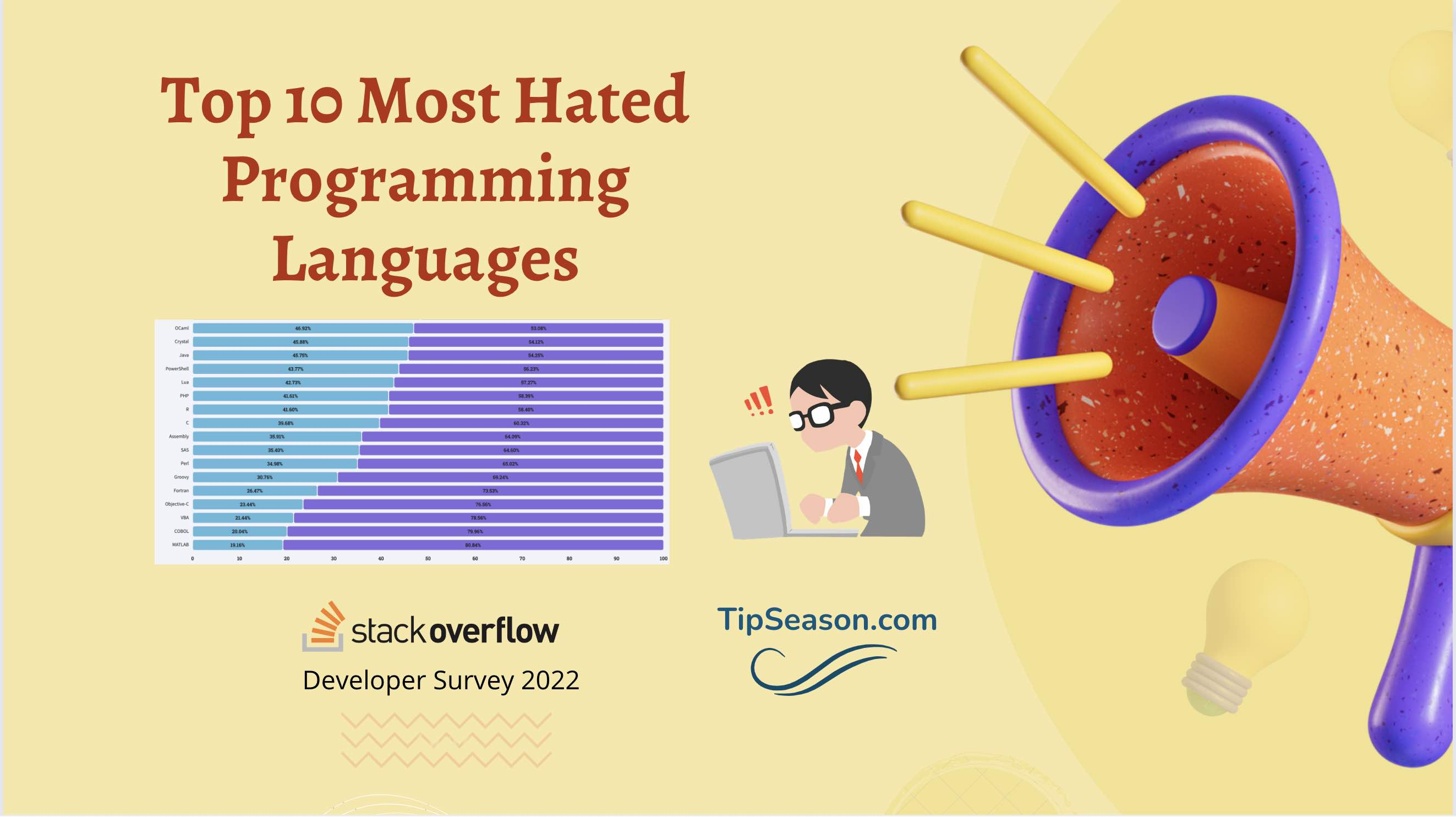# Blind 75 leetcode list - Questions & SolutionsMani
Educating everyone with the beauty of programming!!Here is the list of top 10 most hated programming languages in 2022 and what are they used for!

## 1. Two Sum

#### Problem

Given an array of integers `nums` and an integer `target`, return indices of the two numbers such that they add up to `target`.

You may assume that each input would have exactly one solution, and you may not use the same element twice.

You can return the answer in any order.

Example 1:

```Input: nums = [2,7,11,15], target = 9
Output: [0,1]
Explanation: Because nums + nums == 9, we return [0, 1].
```

Example 2:

```Input: nums = [3,2,4], target = 6
Output: [1,2]
```

Example 3:

```Input: nums = [3,3], target = 6
Output: [0,1]
```

Constraints:

• `2 <= nums.length <= 104`
• `-109 <= nums[i] <= 109`
• `-109 <= target <= 109`
• Only one valid answer exists.

#### Solution

``````1
2
3
4
5
6
7
8
9
10
11
12
13
14
class Solution {
public int[] twoSum(int[] a, int target) {
Map<Integer, Integer> map = new HashMap<Integer, Integer>();
for(int i = 0; i< a.length; i++) {
Integer data = map.get(target - a[i]);
if(data == null) {
map.put(a[i], i);
} else {
return new int[]{data, i};
}
}
return null;
}
}
``````

## 2. Best Time to Buy and Sell Stock

#### Problem (Leetcode 121)

You are given an array `prices` where `prices[i]` is the price of a given stock on the `ith` day.

You want to maximize your profit by choosing a single day to buy one stock and choosing a different day in the future to sell that stock.

Return the maximum profit you can achieve from this transaction. If you cannot achieve any profit, return `0`.

Example 1:

```Input: prices = [7,1,5,3,6,4]
Output: 5
Explanation: Buy on day 2 (price = 1) and sell on day 5 (price = 6), profit = 6-1 = 5.
Note that buying on day 2 and selling on day 1 is not allowed because you must buy before you sell.
```

Example 2:

```Input: prices = [7,6,4,3,1]
Output: 0
Explanation: In this case, no transactions are done and the max profit = 0.
```

Constraints:

• `1 <= prices.length <= 105`
• `0 <= prices[i] <= 104`

#### Solution

``````1
2
3
4
5
6
7
8
9
10
11
12
13
14
15
16
17
18
19
20
class Solution {
public int maxProfit(int[] prices) {
int max = 0;
if(prices.length == 0) {
return max;
}
int min = prices;
for(int i = 0; i< prices.length; i++) {
int val = prices[i] - min;
if(val > max) {
max = val;
}
if(min > prices[i]) {
min = prices[i];
}
}
return max;
}
}

``````